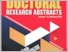# Modified artificial neural network (ANN) models for Malaysian construction costs indices (MCCI) data / Saadi Ahmad Kamaruddin

Ahmad Kamaruddin, Saadi (2018) Modified artificial neural network (ANN) models for Malaysian construction costs indices (MCCI) data / Saadi Ahmad Kamaruddin. In: The Doctoral Research Abstracts. IGS Biannual Publication, 14 . Institute of Graduate Studies, UiTM, Shah Alam.

## Abstract

Artificial neural network (ANN) is one of the most prominent universal approximators, and has been implemented tremendously in forecasting arena. The aforementioned neural network forecasting models are feedforward (nonlinear autoregressive) and recurrent (nonlinear autoregressive moving average). Theoretically, the most common algorithm to train the network is the backpropagation (BP) algorithm which is based on the minimization of the ordinary least squares (LS) estimator in terms of mean squared error (MSE). However, this algorithm is not totally robust in the presence of outliers that usually exist in the routine time series data, and this may cause false prediction of future values. Therefore, the main objective of this research is to modify the backpropagation algorithm of nonlinear autoregressive (NAR) and autoregressive moving average (NARMA) models using Tukey-bisquare estimator and a proposed hybrid firefly algorithm on the least median of squares (FFA-LMedS), in order to manage outlying data efficiently, hence produce more accurate forecasted values. The proposed neural network models are named as modified NAR and NARMA models, which able to handle various degrees of outliers problem in time series data. The performance of the fitted neural network models are examined on both real and simulated datasets. The error measures to assess the performance are Root Mean Square Errors (RMSE), Mean Square Prediction Error (MSPE), Mean Absolute Percentage Error (MAPE), Mean Absolute Deviation (MAD) and Geometric Root Mean Square Error (GRMSE)…

Item Type: Book Section
Creators:
CreatorsID Num. / Email
Subjects: Q Science > QA Mathematics > Analysis
Q Science > QA Mathematics > Instruments and machines
Divisions: Universiti Teknologi MARA, Shah Alam > Institut Pengajian Siswazah (IPSis) : Institute of Graduate Studies (IGS)
Series Name: IGS Biannual Publication
Volume: 14
Item ID: 22104
Uncontrolled Keywords: Abstract; Abstract of thesis; Newsletter; Research information; Doctoral graduates; IPSis; IGS; UiTM; artificial neural network
URI: http://ir.uitm.edu.my/id/eprint/22104Preview
Text (ABSTRACT ONLY)View Item